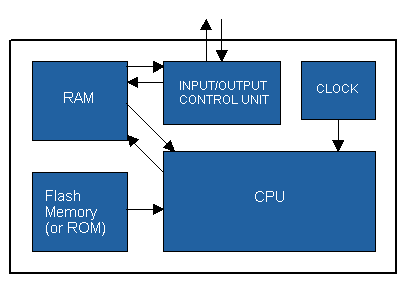# block diagram

Also found in: Dictionary, Thesaurus, Financial, Acronyms, Wikipedia.

## block diagram

[′bläk ‚dī·ə‚gram]
(engineering)
A diagram in which the essential units of any system are drawn in the form of rectangles or blocks and their relation to each other is indicated by appropriate connecting lines.

## Block diagram

A convenient graphical representation of input-output behavior of a system, where the signal into the block represents the input and the signal out of the block represents the output. The flow of information (the signal) is unidirectional from the input to the output. The primary use of the block diagram is to portray the interrelationship of distinct parts of the system.

A block diagram consists of two basic functional units that represent system operations. The individual block symbols portray the dynamic relations between the input and output signals. The second type of unit, called a summing point, is represented by a circle with arrows feeding into it. The operation that results is a linear combination of incoming signals to generate the output signal. The sign appearing alongside each input to the summing point indicates the sign of that signal as it appears in the output.

Block diagrams are widely used in all fields of engineering, management science, criminal justice, economics, and the physical sciences for the modeling and analysis of systems. In modeling a system, some parameters are first defined and equations governing system behavior are obtained. A block diagram is constructed, and the transfer function for the whole system is determined.

If a system has two or more input variables and two or more output variables, simultaneous equations for the output variables can be written. In general, when the number of inputs and outputs is large, the simultaneous equations are written in matrix form.

Block diagrams can be used to portray nonlinear as well as linear systems, such as a cascade containing a nonlinear amplifier and a motor. See Computer programming, Gain, Systems engineering

## block diagram

A chart that contains squares and rectangles connected with arrows to depict hardware and software interconnections. For program flow charts, information system flow charts, circuit diagrams and communications networks, more elaborate graphical representations are usually used.

Block Diagram of a ComputerReferences in periodicals archive ?
Caption: Figure 5: The block diagram of the hybrid frequency synthesizer with DDS as a support oscillator of the PLL.
Through the block diagrams in Simulink environments we are able to simulate the system response to various experimental situations.
Some parts of Rhapsody model are designated as Simulink blocks: their detailed behavior is described using Simulink block diagrams. The user generates C/C++ code from the corresponding Simulink models by using RealTime Workshop Embedded Coder.
System reliability using reliability block diagrams (RBD)
The more work applied to the block diagram, concentrating on clear, intuitive, visual work signals and balance, the easier the actual scaled physical layout will be--and the more Lean Flow benefits the actual new manufacturing layout will provide.
Figure 3 shows block diagram of a MIMO OFDM system with 2 transmit and 2 receive antennas according to which simulation is done.
A script node is a special edit node place on the block diagram of the VI in which you can type command statements in the language chosen for that instance of the script node, in this case IDL.
In a typical board-level thermal simulation process flow, the systems architect will develop the initial concept design by creating a functional block diagram. The hardware design engineer then derives the first physical layout directly from the block diagram.
1), the energy transmission system is an interface between an input (prime mover) and an output (load).The energy transmission system must next be broken down into its functional sections, as shown in the block diagram (Fig.
The controller's standard CoDeSys IEC 61131-3 programming environment enables programming in any one of six different languages including: Structured Text (ST), Function Block Diagram (FBD), Ladder Diagram (LD), Instruction List (IL), Sequential Function Chart (SFC), and Continuous Function Chart (CFC), so engineers can program in whatever language they prefer.

Site: Follow: Share:
Open / Close